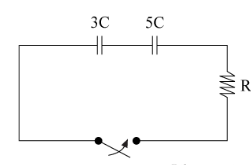# Problem: Two capacitors of capacitance 3C and 5C (where C = 0.13 F) are connected in series with a resistor of resistance R Randomized Variables R=5.5 Ω How long will it take the amount of charge in the circuit to drop by 75% in seconds?

###### FREE Expert Solution

Equivalent capacitance for two capacitors:

$\overline{){{\mathbf{C}}}_{\mathbf{e}\mathbf{q}}{\mathbf{=}}\frac{{\mathbf{C}}_{\mathbf{1}}{\mathbf{C}}_{\mathbf{2}}}{{\mathbf{C}}_{\mathbf{1}}\mathbf{+}{\mathbf{C}}_{\mathbf{2}}}}$

The time constant for RC circuits:

$\overline{){\mathbf{\tau }}{\mathbf{=}}{\mathbf{R}}{\mathbf{C}}}$

Discharging a capacitor in RC circuits:

$\overline{){\mathbf{Q}}{\mathbf{=}}{{\mathbf{Q}}}_{{\mathbf{0}}}{\mathbf{\left(}}{\mathbf{1}}{\mathbf{-}}{{\mathbf{e}}}^{\mathbf{-}\mathbf{t}\mathbf{/}\mathbf{\tau }}{\mathbf{\right)}}}$

Ceq = (C1C2)/(C1 + C2) = (3C * 5C)/(3C + 5C) = (15/8)C = (15/8)0.13 = 0.24375 F

85% (24 ratings)###### Problem Details

Two capacitors of capacitance 3C and 5C (where C = 0.13 F) are connected in series with a resistor of resistance R Randomized Variables R=5.5 ΩHow long will it take the amount of charge in the circuit to drop by 75% in seconds?